## Matrix Range

A linear system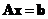can be thought as a linear transformation of a vector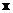in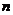dimensional space into vector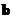in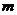dimensional space. The solution spaceof a non-homogeneous system of equationsis called the range of matrix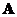and the dimension of the range of matrixis called the rank ofsymbolizes as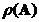.

The dimension of the range and the null space of a matrix are related through fundamental relationship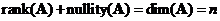. Whereis the number of original unknowns.